# Multiplying Fractions and Area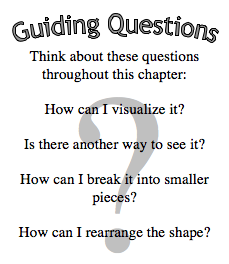In Section 5.1, you will calculate portions of fractions, or “parts of parts.”  You will use these ideas to develop strategies for multiplying fractions and mixed numbers.

In Section 5.2, your new knowledge of multiplying fractions will make it possible for you to make sense of decimal multiplication.  You will also investigate how multiplying by a number close to, much larger than, or much smaller than one affects size of the product.

Section 5.3 focuses on the question, “How can we use what we know about the area of basic shapes to find the area of complex shapes?”  As you develop new strategies for finding the area of shapes, you will be able to solve new problems that involve more complex areas.

In this chapter, you will learn:

• How to calculate a part of another part.
• How to multiply fractions, mixed numbers, and decimals.
• Find the area of shapes, including rectangles, triangles, parallelograms, and trapezoids.
• Break complex shapes into smaller pieces in order to find area.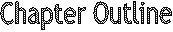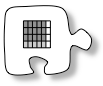Section 5.1    You will learn how to multiply fractions by examining portions of fractions.  You will also connect this process to finding the products of mixed numbers.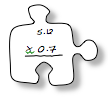Section 5.2    In the second section, you will extend what you learned in the first section to find products of decimals and to understand how multiplication by numbers greater than or less than one affects the product.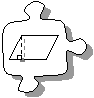Section 5.3    You will find the area of different shapes such as parallelograms, triangles, and trapezoids by rearranging them into rectangles.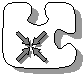Section 5.4    Finally, you will reflect on the content and work you have done in Chapters 1 through 5.

### WHAT TOOLS CAN I USE?

• You have several tools and references available to help support your learning: your teacher, your study team, your math book, and your Toolkit, to name only a few.  At the end of each chapter, you will have an opportunity to review your Toolkit for completeness.  You will also revise or update it to reflect your current understanding of big ideas.
• The main elements of your Toolkit should be your Learning Log, Math Notes, and the vocabulary used in this chapter.  Math words that are new appear in bold in the text.  Refer to the lists provided below and follow your teacher’s instructions to revise your Toolkit, which will help make it useful for you as you complete this chapter and as you work in future chapters.
• Learning Log Entries• Math Notes

## Mathematical Vocabulary

• The following is a list of vocabulary found in this chapter.  Some of the words have been seen in a previous chapter.  The italicized words are new to this chapter.  Make sure that you are familiar with the terms below and know what they mean.  Click on the word for a “pop-up” definition. For more information, refer to the glossary or index.  You might also add these words to your Toolkit so that you can reference them in the future.
•  area base dimensions generic rectangle height mixed numbers parallel perpendicular product quadrilateral ratio trapezoid triangle